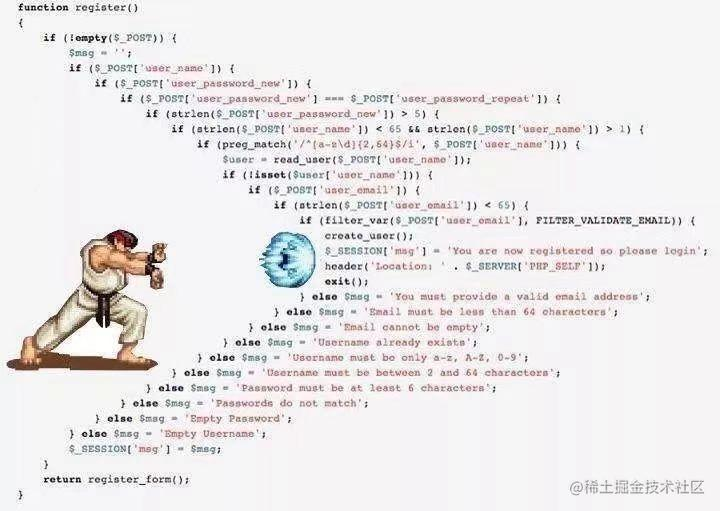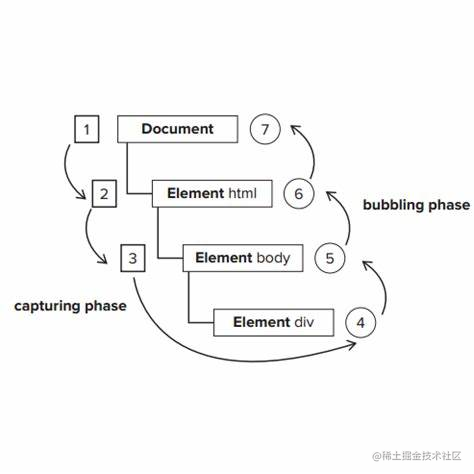# 如何避免使用过多的 if else？

## 一、引言## 二、8种`if-else`的优化/替代方案

### 1. 使用排非策略：!、!!

``````1、判断是否为空if(value === null || value === NaN || value === 0 || value === ''|| value === undefined   )
{
……
}2、判断是否数组是否含有符合某条件的元素const name = arr.find(item => item.status === 'error')?.name;if(name !== undefined && name !== ''){
……
}``````

``1、判断是否为空if(!value){……}2、判断是否数组是否含有符合某条件的元素if(!!arr.find(item => item.status === 'error')?.name){……}``

### 2. 使用条件（三元）运算符： c ? t : f

condition ? exprIfTrue : exprIfFalse；

``````let beverage = '';if(age > 20){
beverage = 'beer';
} else {
beverage = 'juice';
}``````

``const beverage = age > 20 ? 'beer' : 'juice';``

` tips: 建议只用一层三元运算符，多层嵌套可读性差。`

### 3. 使用短路运算符：&&、 ||

• `&&` 为取假运算，从左到右依次判断，如果遇到一个假值，就返回假值，以后不再执行，否则返回最后一个真值；
• `||` 为取真运算，从左到右依次判断，如果遇到一个真值，就返回真值，以后不再执行，否则返回最后一个假值。

``````    if (isOnline){makeReservation(user);
}``````

`` isOnline && makeReservation(user);``

### 4. 使用 switch 语句

``````    let result;if (type === 'add'){
result = a + b;
} else if(type === 'subtract'){
result = a - b;
} else if(type === 'multiply'){
result = a * b;
} else if(type === 'divide'){
result = a / b;
} else {console.log('Calculation is not recognized');
}``````

``````let result;switch (type) {case 'add':
result = a + b;break;case 'subtract':
result = a - b;break;case 'multiply':
result = a * b;break;case 'divide':
result = a / b;break;default:console.log('Calculation is not recognized');
}``````

### 5. 定义相关函数拆分逻辑，简化代码

``````function itemDropped(item, location) {if (!item) {return false;
} else if (outOfBounds(location) {var error = outOfBounds;
server.notify(item, error);
items.resetAll();return false;
} else {animateCanvas();
server.notify(item, location);return true;
}
}``````

``````// 定义dropOut和dropIn, 拆分逻辑并提高代码可读性function itemDropped(item, location) {const dropOut = function () {
server.notify(item, outOfBounds);
items.resetAll();return false;
};const dropIn = function () {animateCanvas();
server.notify(item, location);return true;
};return !!item && (outOfBounds(location) ? dropOut() : dropIn());
}``````

### 6. 将函数定义为对象，通过穷举查找对应的处理方法

• 定义普通对象

对于方案3的例子，不妨尝试这么写：

``````function calculate(action, num1, num2) {
const actions = {
add: (a, b) => a + b,
subtract: (a, b) => a - b,
multiply: (a, b) => a * b,
divide: (a, b) => a / b,
};
​
return actions[action]?.(num1, num2) ?? "Calculation is not recognized";
}``````

• 定义 Map 对象

普通对象的键需要是字符串，而 Map 对象的键可以是一个对象、数组或者更多类型，更加灵活。

``````let statusMap = new Map([
[
{ role: "打工人", status: "1" },() => { /*一些操作*/},
],
[
{ role: "打工人", status: "2" },() => { /*一些操作*/},
],
[
{ role: "老板娘", status: "1" },() => { /*一些操作*/},
],
]);let getStatus = function (role, status) {
statusMap.forEach((value, key) => {if (JSON.stringify(key) === JSON.stringify({ role, status })) {value();
}
});
};getStatus("打工人", "1"); // 一些操作``````

tips: JSON.stringify()可用于深比较/深拷贝。

### 7. 使用责任链模式``````function demo (a, b, c) {if (f(a, b, c)) {if (g(a, b, c)) {// ...
}// ...else if (h(a, b, c)) {// ...
}// ...
} else if (j(a, b, c)) {// ...
} else if (k(a, b, c)) {// ...
}
}``````

``````const rules = [
{match: function (a, b, c) { /* ... */ },action: function (a, b, c) { /* ... */ }
},
{match: function (a, b, c) { /* ... */ },action: function (a, b, c) { /* ... */ }
},
{match: function (a, b, c) { /* ... */ },action: function (a, b, c) { /* ... */ }
}// ...
]// 每个职责一旦匹配，原函数就会直接返回。function demo (a, b, c) {for (let i = 0; i < rules.length; i++) {if (rules[i].match(a, b, c)) {return rules[i].action(a, b, c)
}
}
}``````

## 三、小结